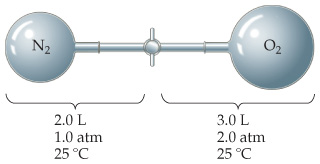# Problem: Consider the apparatus shown in the drawing.What is the partial pressure of O2 in the mixture?

###### FREE Expert Solution

Recall that the ideal gas law is:

$\overline{){\mathbf{PV}}{\mathbf{=}}{\mathbf{nRT}}}$

82% (12 ratings)###### Problem Details

Consider the apparatus shown in the drawing.

What is the partial pressure of O2 in the mixture?

Frequently Asked Questions

What scientific concept do you need to know in order to solve this problem?

Our tutors have indicated that to solve this problem you will need to apply the The Ideal Gas Law Derivations concept. You can view video lessons to learn The Ideal Gas Law Derivations. Or if you need more The Ideal Gas Law Derivations practice, you can also practice The Ideal Gas Law Derivations practice problems.